# Equilateral Triangle

Here we will learn about equilateral triangles, including what an equilateral triangle is and how to solve problems involving the sides and angles of equilateral triangles.

There are also equilateral triangles worksheets based on Edexcel, AQA and OCR exam questions, along with further guidance on where to go next if you’re still stuck.

## What is an equilateral triangle?

An equilateral triangle is a type of triangle that has the following properties,

• All sides are equal length.

• All angles are equal size (60^{\circ}) .

An equilateral triangle is the simplest regular polygon.

All equilateral triangles are similar to (an enlargement of) each other as they have the same angles.

Equilateral triangles are only congruent if the lengths of the sides are the same.

An equilateral triangle is a special case of an acute triangle, where all the angles are acute angles.

Properties of equilateral triangles and other types of triangles are very important within geometry.

They are one of the most common shapes to recognise for angles in parallel lines, circle theorems, interior angles, trigonometry, Pythagoras’ theorem and many more. You therefore must be familiar with their individual properties.

### What is an equilateral triangle?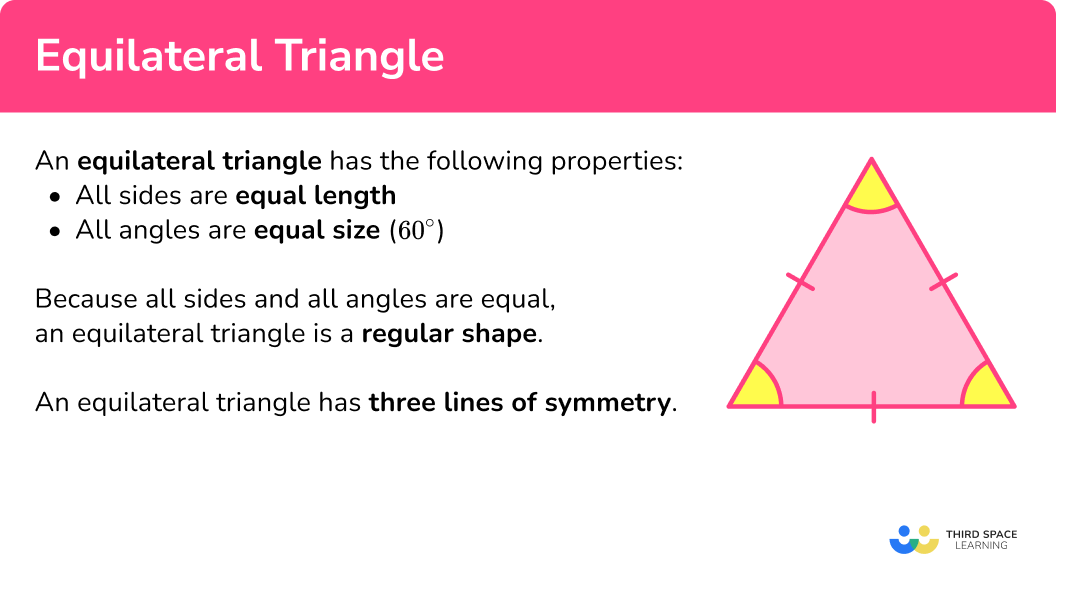### Symmetry

An equilateral triangle has three lines of symmetry. The lines go from one vertex to the middle of the opposite side.

The lines of symmetry are angle bisectors of the vertices.

The lines of symmetry are also perpendicular bisectors of the sides of the equilateral triangle.

An equilateral triangle has an order of rotational symmetry of 3 as it looks the same three times in a full turn.

In other words, if you rotate an equilateral triangle about its centre, it looks like the original every 120^{\circ} rotation clockwise.

### Area of an equilateral triangle

The area of an equilateral triangle can be found by using the formula A=\cfrac{1}{2} \, bh, where b is the base length and h is the perpendicular height of an equilateral triangle. Sometimes these values need to be calculated.

Remember that an area uses square units.

For example, calculate the area of the equilateral triangle ABC below, where M is the midpoint of the line AC , and angle AMB = 90^{\circ} .

Here, the base AC is double the length of AM and so AC = 8{~cm} .

Substituting the base length b = 8 and the perpendicular height h = 6.92 into the formula for the area of a triangle, we have

A=\cfrac{1}{2}\times{8}\times{6.92}= 27.68\text{~cm}^{2}.

The area of the triangle is 27.68 \, cm^2.

Step-by-step guide: Area of an equilateral triangle

### 3D shapes

A regular tetrahedron can be made by using 4 equilateral triangles.

An octahedron can be made by using 8 equilateral triangles.

An icosahedron can be made by using 20 equilateral triangles.

Step-by-step guide: 3D shape names

## How to solve problems involving equilateral triangles

In order to solve problems involving equilateral triangles:

1. Locate known angles and calculate any necessary unknown angles.
2. Locate known sides and calculate any necessary unknown side lengths.
3. Solve the problem using any necessary values.

### Explain how to solve problems involving equilateral triangles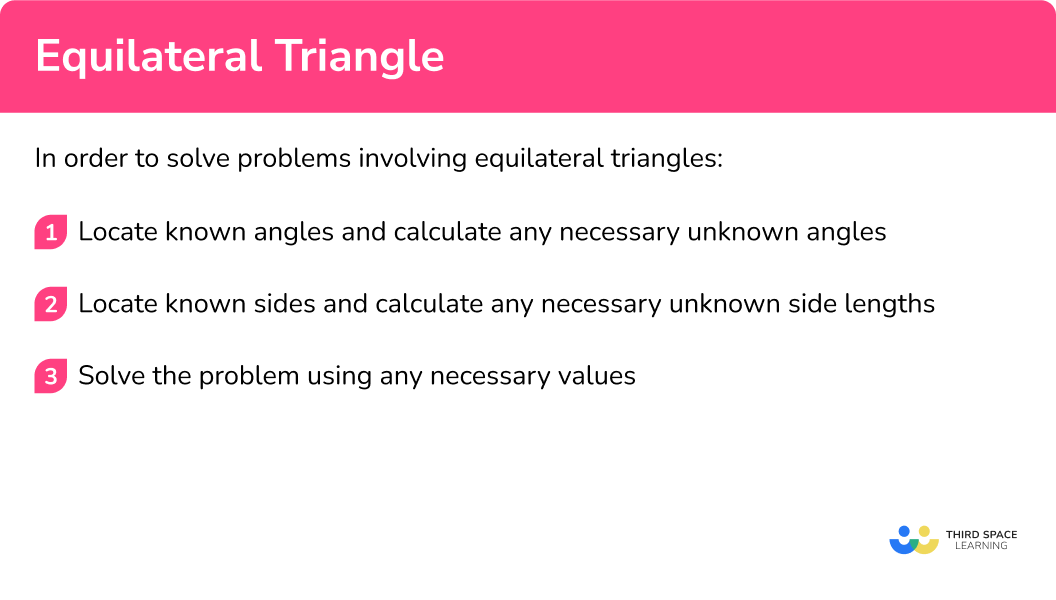### Related lessons on triangles

Equilateral triangle is part of our series of lessons to support revision on polygons and triangles. You may find it helpful to start with the main triangles lesson for a summary of what to expect, or use the step by step guides below for further detail on individual topics. Other lessons in this series include:

## Equilateral triangle examples

### Example 1: perimeter of an equilateral triangle

Find the perimeter of an equilateral triangle with side 7{~cm} .

1. Locate known angles and calculate any necessary unknown angles.

We do not need to calculate any angles as the perimeter is a measure of length and we can determine the side lengths of the triangle from the information provided.

2Locate known sides and calculate any necessary unknown side lengths.

As the triangle is an equilateral triangle, all three side lengths are the same and are equal to 7{~cm} .

3Solve the problem using any necessary values.

We can calculate the perimeter by multiplying one of the side lengths by 3 (or adding the three side lengths), 3\times 7=21 .

The perimeter of the equilateral triangle is 21{~cm} .

### Example 2: perimeter of a 2D compound shape

Find the perimeter of this trapezium made from congruent equilateral triangles with side 20{~cm} .

As each triangle in the diagram is an equilateral triangle (stated in the question), we know that each interior angle is 60^{\circ} . However, this information is not needed for this problem.

As each side length of an equilateral triangle is the same, each side can be labelled as 20{~cm} although, we do not need to label the two lines within the shape as these are not needed for the perimeter.

The perimeter of the shape is calculated by adding up all of the external side lengths.

20+20+20+20+20=100\text{~cm}

The perimeter of the compound shape is 100{~cm} (or 1{~m} ).

### Example 3: external angles of an equilateral triangle

Calculate the size of angle x .

Each side length of the triangle contains a dash and so the triangle is equilateral. This means that all interior angles are 60^{\circ} .

As we want to calculate the size of the missing angle, we do not need to know any side lengths to help us find the value of x .

Adjacent angles on a straight line add up to 180^{\circ} and so x=180-60=120^{\circ} .

Therefore angle x=120^{\circ} .

### Example 4: similar equilateral triangles problem solving

An equilateral triangle ABC has a perimeter of 30{~cm} .

Points M and N are midpoints of the lines AB and AC respectively where MN is parallel to BC . Show that the line MN = 5{~cm} .

As ABC is an equilateral triangle, each interior angle is equal to 60^{\circ} .

As corresponding angles in parallel lines are equal, and angle AMN is corresponding to angle ABC , angle AMN = 60^{\circ} .

Also, as angle ANM is corresponding to angle ACB , angle ANM = 60^{\circ} .

We can now see that triangle AMN is also equilateral.

The perimeter of ABC is 30{~cm} , and so each side length must be equal to

30\div{3}=10\text{~cm}.

M is a midpoint of the side AB and so as the length of AB = 10{~cm} , the length of AM is half the length of AB . This means that AM = 5{~cm} .

N is a midpoint of the side AC and so as the length of AC = 10{~cm} , the length of AN is half the length of AC . This means that AN = 5{~cm} .

Triangle AMN is equilateral and so each side length is equal to 5{~cm} .

This means that MN = 5{~cm} .

### Example 5: equilateral triangles and regular hexagons problem solving

Six congruent equilateral triangles are placed next to each other to form a hexagon ABCDEF . The side length of a triangle is 3{~cm} . Show that the hexagon is a regular hexagon.

As each triangle is equilateral, each angle within the triangles is 60^{\circ} . Two angles that meet at the perimeter of the hexagon must therefore have a sum of 60+60=120^{\circ} . Labelling the diagram with this information, we have

We are also told that the side length of each equilateral triangle is 3{~cm} , and so labelling each external side length of ABCDEF , we have

We now know that all of the interior angles of ABCDEF are equal to 120^{\circ} and so they are the same, and each side length of the hexagon is equal to 3{~cm} .

The hexagon is therefore a regular hexagon.

### Example 6: bearings problem with equilateral triangles problem solving

Three planes set off from an airfield at the same height.

Plane A travels at a bearing of 020^{\circ} , Plane B travels at a bearing of 140^{\circ} .

Plane C travels at a bearing of 260^{\circ} . Each plane travels at the same speed.

After 30 minutes, each plane is exactly D{~km} from the airport.

A diagram of this is shown below.

Without calculating the exact value, show that the planes are all the same distance apart from each other

Bearings are always taken from North in a clockwise direction. This means that we can work out the exact angle the planes are on with respect to the airfield.

The angle AOB = 140-20=120^{\circ}.

The angle BOC = 260-140=120^{\circ}.

The angle AOC = (360-260)+20=120^{\circ}.

Sketching these onto a separate diagram, we have

As we are showing that each plane is the same distance apart from each other, we need to show that the length AB = BC = AC and we only know the lengths OA = OB = OC = D{~km} and so we need to do more calculations with angles to show that the planes are the same distance apart.

Let us assume that the distance BC = x{~km} .

This means that OBC is an isosceles triangle as the lines OB and OC are the same length. The angles OBC and OCB must now be equal as they are opposite the two equal side lengths.

As the sum of angles in a triangle is 180^{\circ} , the remaining two angles are half of the difference between 120^{\circ} and 180^{\circ} .

This means that the angles OBC and OCB are equal to (180-120)\div{2}=30^{\circ}.

Repeating this process for triangles AOB and AOC , we have exactly the same result.

Looking at the angles ABC, BCA and CAB , each of these is equal to 30+30=60^{\circ} and as the value of x{~km} is the distance between each plane, ABC is an equilateral triangle, and so all of the planes are the same distance away from each other.

### Common misconceptions

• Angles in polygons
Make sure you know your angle properties. Getting these confused causes quite a few misconceptions.
• Angles in a triangle total 180^{\circ} .
• Angles in a quadrilateral total 360^{\circ} .

• Angle facts
Make sure you know your angle facts.
• Adjacent angles on a straight line add up to 180^{\circ} .
• Vertically opposite angles are equal.
• Alternate angles are equal.
• Corresponding angles are equal.

### Practice equilateral triangle questions

1) Which of these diagrams shows an equilateral triangle?The marks on the sides of the triangle indicate which lengths are equal. We need the triangle which has the same mark on all three sides.

2) How many lines of symmetry does an equilateral triangle have?

1236Equilateral triangles have 3 lines of symmetry that bisect an angle and the opposite side to the vertex.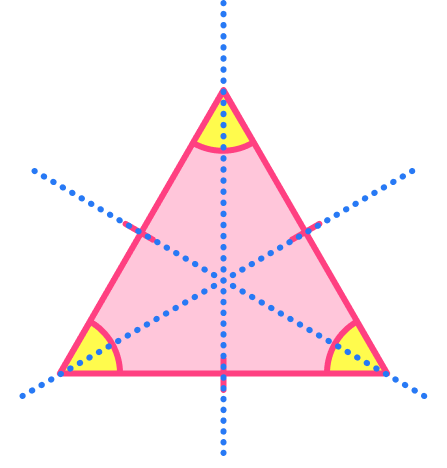3) What is the interior angle of an equilateral triangle?

360^{\circ}50^{\circ}180^{\circ}60^{\circ}The sum of angles in any triangle is 180^{\circ} . To find one interior angle of this regular shape, we can simply divide 180 by 3 to get 180\div 3=60^{\circ}.

4) Calculate the perimeter of an equilateral triangle with sides 3.5{~cm} .

7{~cm}10.5{~cm}6.5{~cm}14{~cm}The perimeter of an equilateral triangle can be found by multiplying the length of one side of the equilateral triangle by 3 .

3.5\times 3=10.5

5) This parallelogram is made from 2 congruent equilateral triangles. Find angle BCD .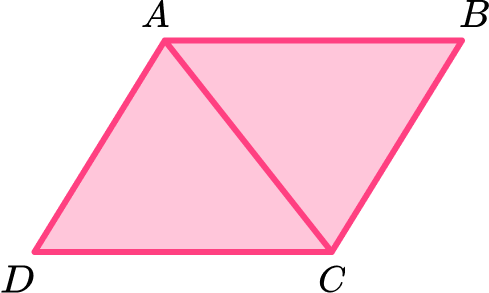120^{\circ}60^{\circ}90^{\circ}180^{\circ}The angle can be found by multiplying the size of one angle in an equilateral triangle by 2 .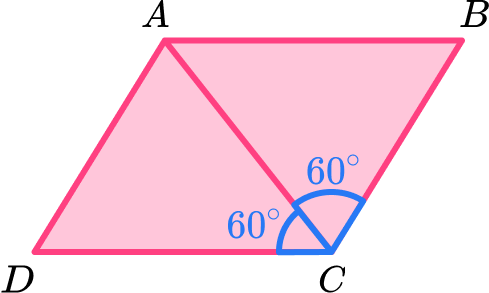2\times 60= 120^{\circ}

6) This 2D shape has been made with 4 congruent triangles with side 12{~cm} . Find the perimeter of the shape.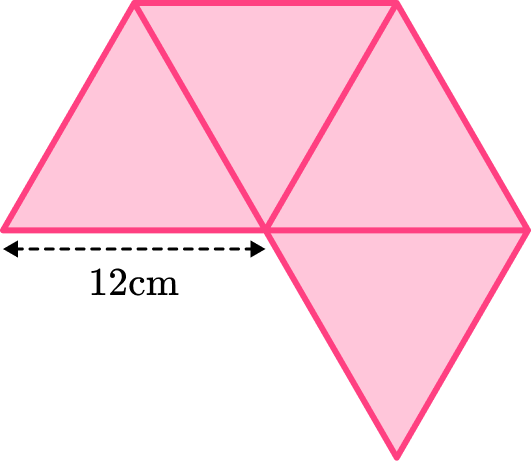144{~cm}120{~cm}72{~cm}84{~cm}The perimeter of the shape can be found by multiplying the length of one side of the equilateral triangle by 6 .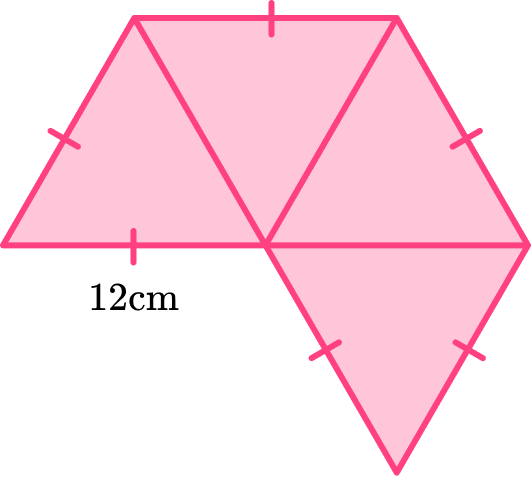12\times 6=72

### Equilateral triangle GCSE questions

1. Shape A is a triangle.Circle the type of triangle it is.

Isosceles Scalene Equilateral Right-angled

(1 mark)

Equilateral

(1)

2. This parallelogram has been made using 4 congruent equilateral triangles with side length 5{~cm} .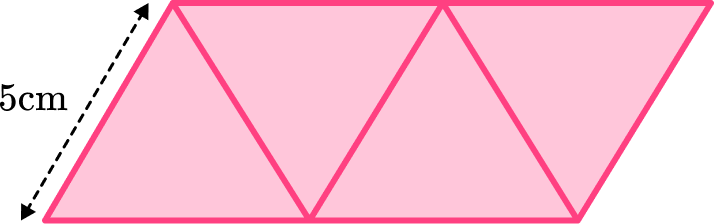Calculate the perimeter of the shape.

(3 marks)

6 \times 5

(1)

30

(1)

cm

(1)

3. ABC is a straight line.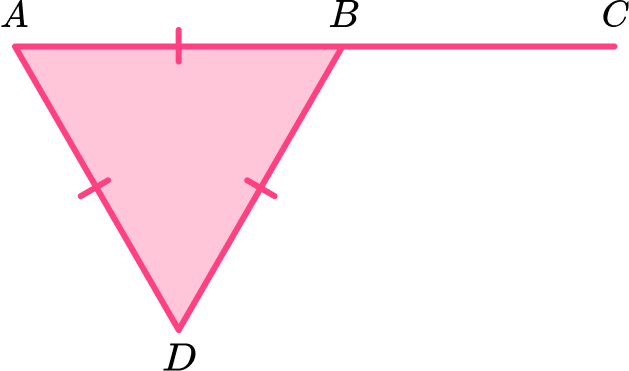CBD = 2x+50^{\circ}

Calculate the value of x .

(4 marks)

ABD= 60^{\circ}

(1)

CBD= 120 ^{\circ}

(1)

2x+50=120 or 2x=70

(1)

x=35

(1)

## Learning checklist

You have now learned how to:

• Recognise an equilateral triangle
• Use the properties of an equilateral triangle to solve problems

## Still stuck?

Prepare your KS4 students for maths GCSEs success with Third Space Learning. Weekly online one to one GCSE maths revision lessons delivered by expert maths tutors.

Find out more about our GCSE maths tuition programme.